PSABM6WU - Student Facing Task---Acc7.5 Lesson 20 Describing Trends in Scatter Plots (8.SP.A.1, 8.SP.A.2)

Which one doesn’t belong?Part A)

Your teacher will give you a piece of pasta and a straightedge.

Here are two copies of the same scatter plot. Experiment with drawing lines to fit the data. Pick the line that you think best fits the data. Compare it with a partner’s.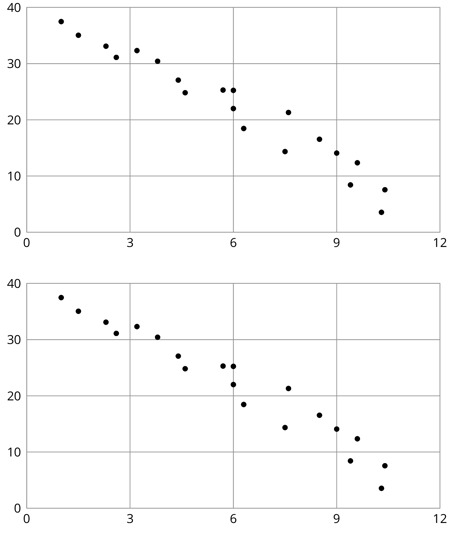Using the graph your teacher provided, draw your line of best fit on paper, take a picture, and upload it using the upload icon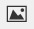If you do not have the ability to upload an image of your work, type "Graph is on paper."

Part B)

Here are two copies of another scatter plot. Experiment with drawing lines to fit the data. Pick the line that you think best fits the data. Compare it with a partner’s.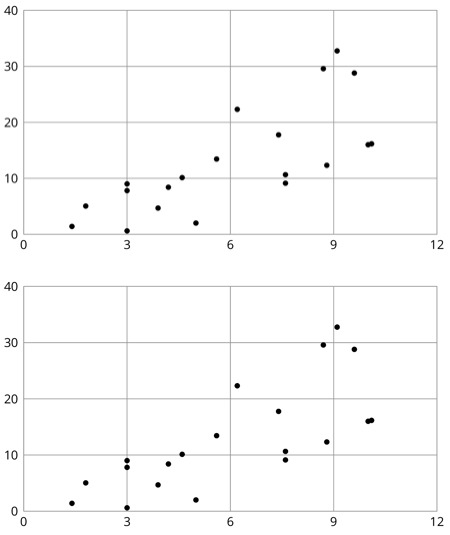Draw your graph on paper, take a picture, and upload it using the upload iconIf you do not have the ability to upload an image of your work, type "Graph is on paper."

Part C)

In your own words, describe what makes a line fit a data set well.

Part A)

The scatter plots both show the year and price for the same 17 used cars. However, each scatter plot shows a different model for the relationship between year and price.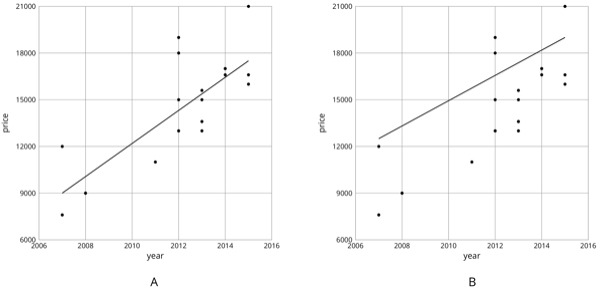Look at Diagram A

For how many cars does the model in Diagram A make a good prediction of its price?

Part B)

Look at Diagram A

For how many cars does the model underestimate the price?

Part C)

Look at Diagram A

For how many cars does the model overestimate the price?

Part D)

The scatter plots both show the year and price for the same 17 used cars. However, each scatter plot shows a different model for the relationship between year and price.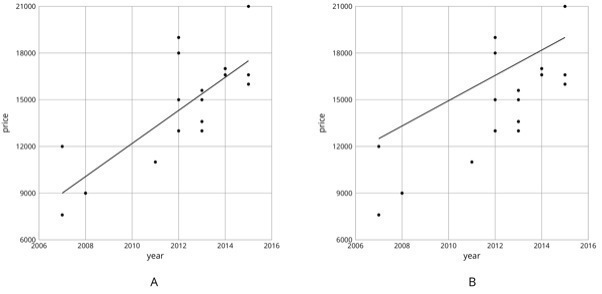Look at Diagram B.

For how many cars does the model in Diagram B make a good prediction of its price?

Part E)

Look at Diagram B.

For how many cars does the model underestimate the price?

Part F)

Look at Diagram B.

For how many cars does the model overestimate the price?

Part G)

For how many cars does the prediction made by the model in Diagram A differ by more than \$3,000? What about the model in Diagram B?

Part H)

Which model does a better job of predicting the price of a used car from its year?

Select one:
Part A)

Is this line a good fit for the data?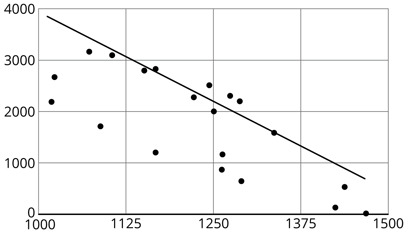Select one:
Part B)

Draw a line that fits the data better.

Using the graph your teacher provided, draw your line of best fit on paper, take a picture, and upload it using the upload icon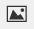If you do not have the ability to upload an image of your work, type "Graph is on paper."

Part C)

Is this line a good fit for the data?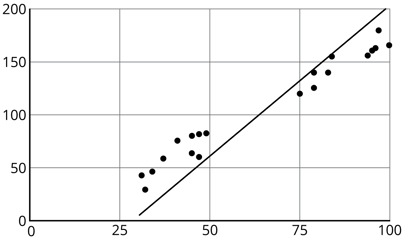Select one:
Part D)

Part E)

Draw a line that fits the data better.

Using the graph your teacher provided, draw your line of best fit on paper, take a picture, and upload it using the upload iconIf you do not have the ability to upload an image of your work, type "Graph is on paper."

Part A)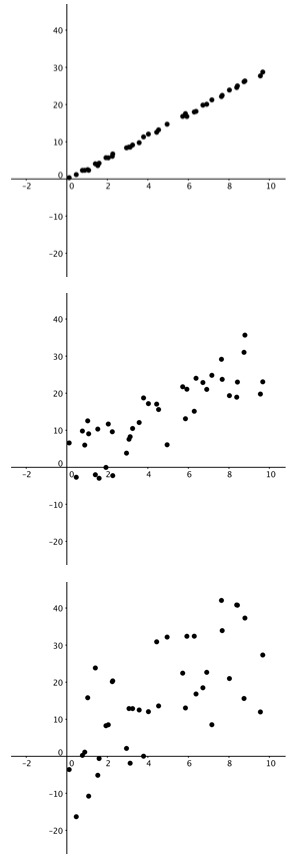These scatter plots were created by multiplying the x-coordinate by 3 then adding a random number between two values to get the y-coordinate
.
The first scatter plot added a random number between -0.5 and 0.5 to the y-coordinate. The second scatter plot added a random number between -2 and 2 to the y-coordinate. The third scatter plot added a random number between -10 and 10 to the y-coordinate.

For each scatter plot, draw a line that fits the data.

Using the graph your teacher provided, draw your line of best fit on paper, take a picture, and upload it using the upload iconIf you do not have the ability to upload an image of your work, type "Graph is on paper."